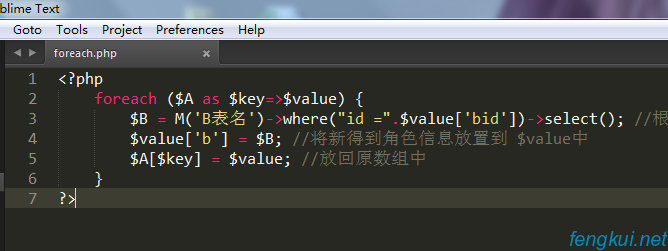# foreach循环

foreach 循环用于遍历数组，例：

``````foreach (\$array as \$value)
{
要执行代码;
}``````

``````<?php
\$x=array("one","two","three");
foreach (\$x as \$value)
{
echo \$value . "<br>";
}
?>``````

``````one
two
three``````

``````foreach (\$A as \$key=>\$value) {
\$B = M('B表名')->where("id =".\$value\['bid'\])->select(); //根据关联的键值查询数据
\$value\['b'\] = \$B; //将新得到角色信息放置到 \$value中
\$A\[\$key\] = \$value; //放回原数组中
}``````